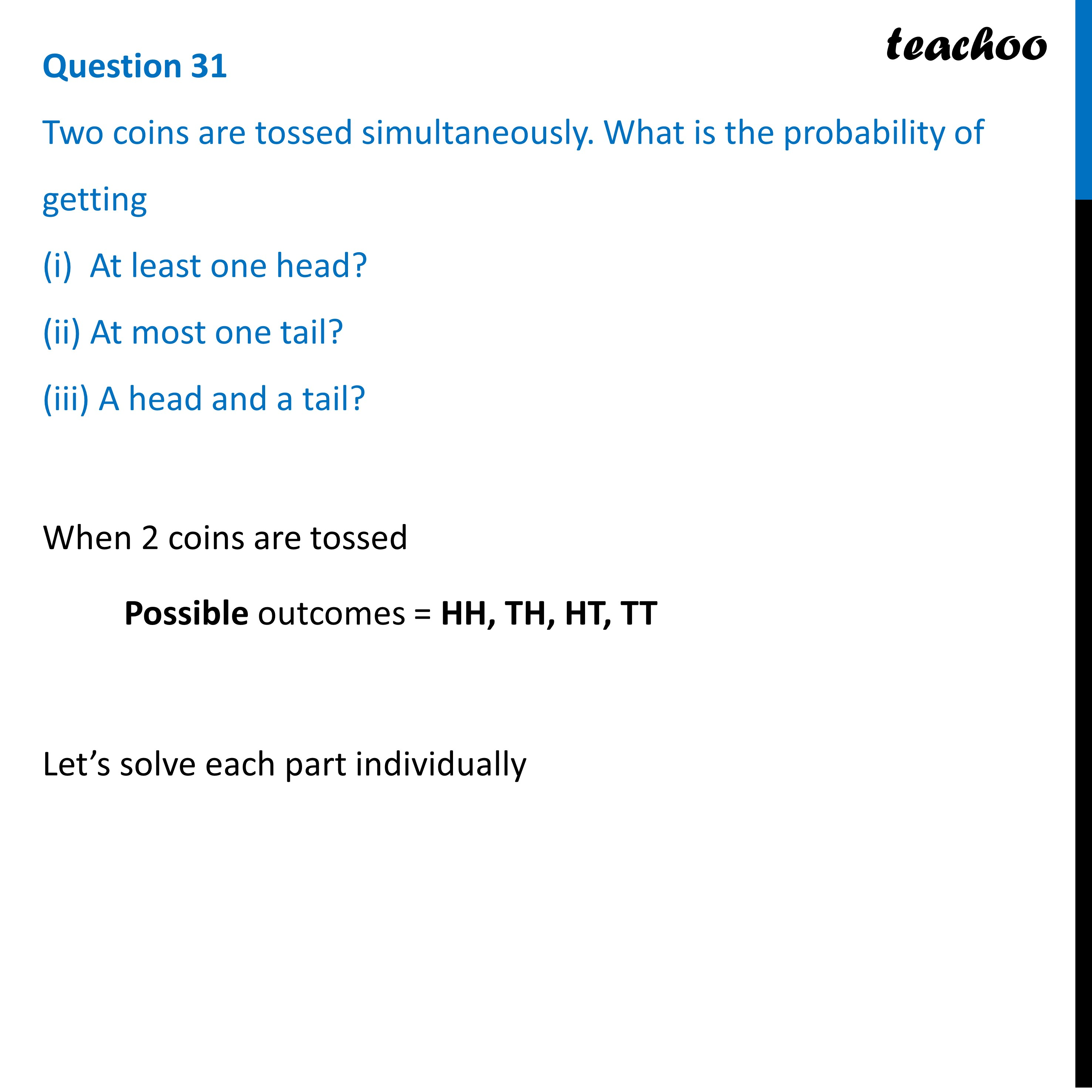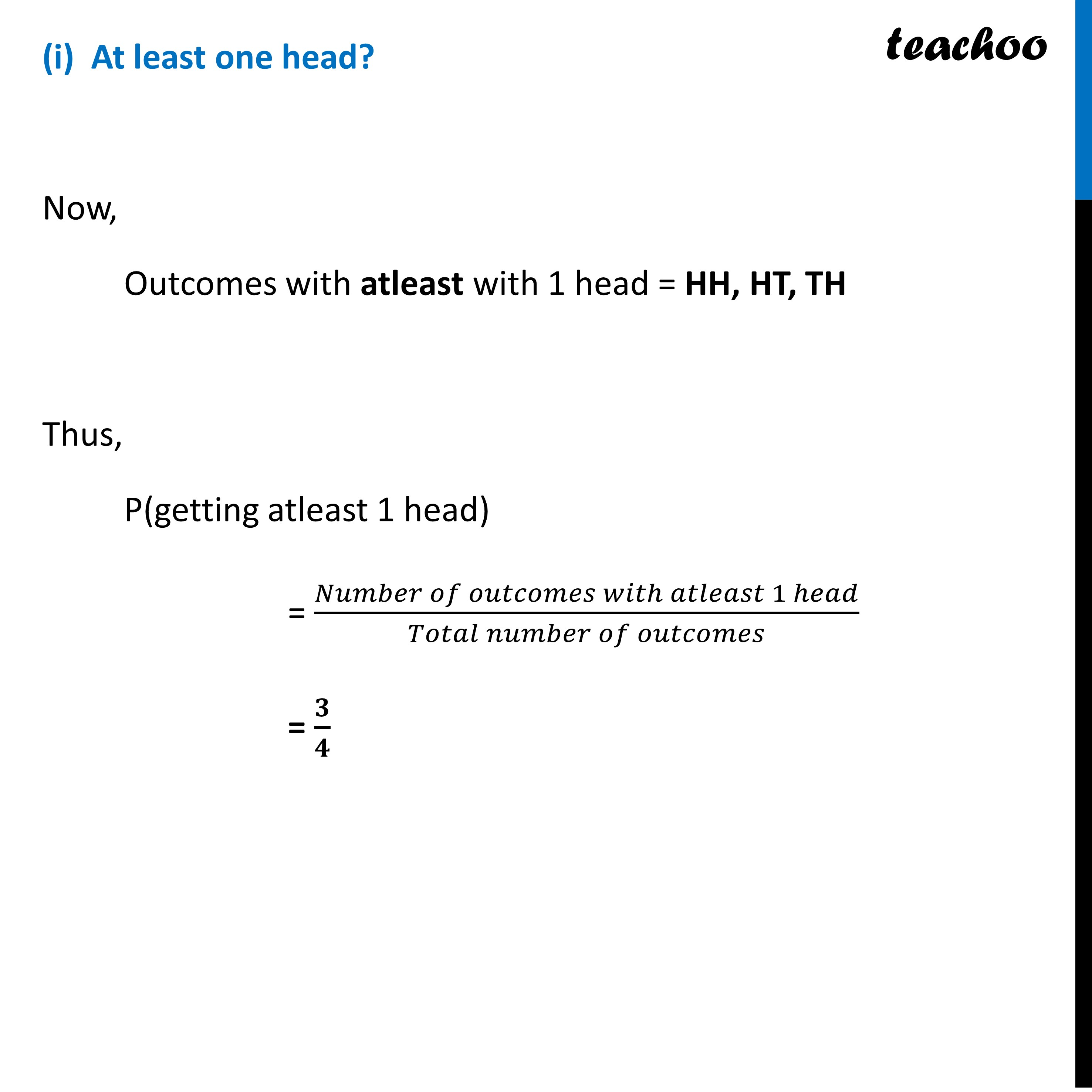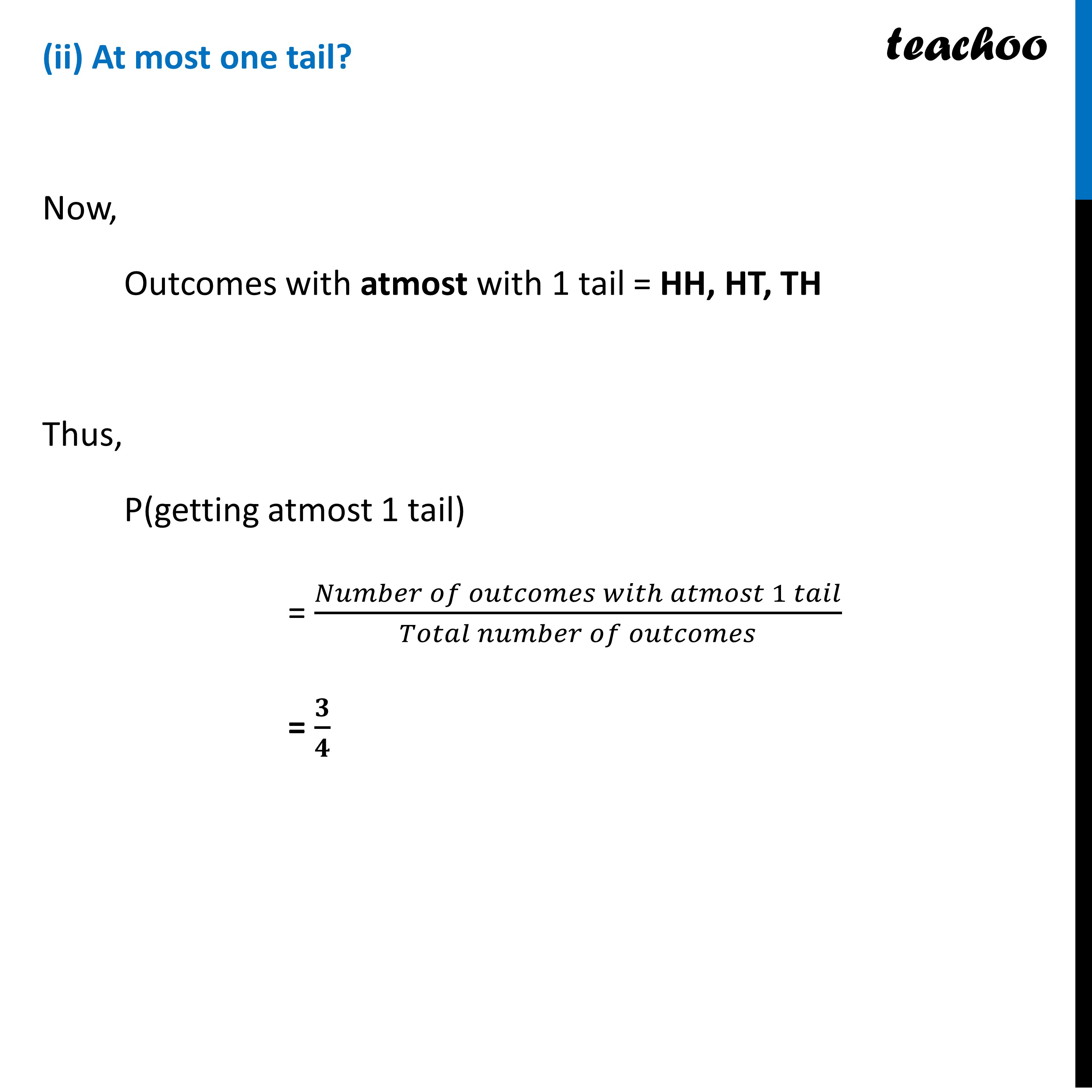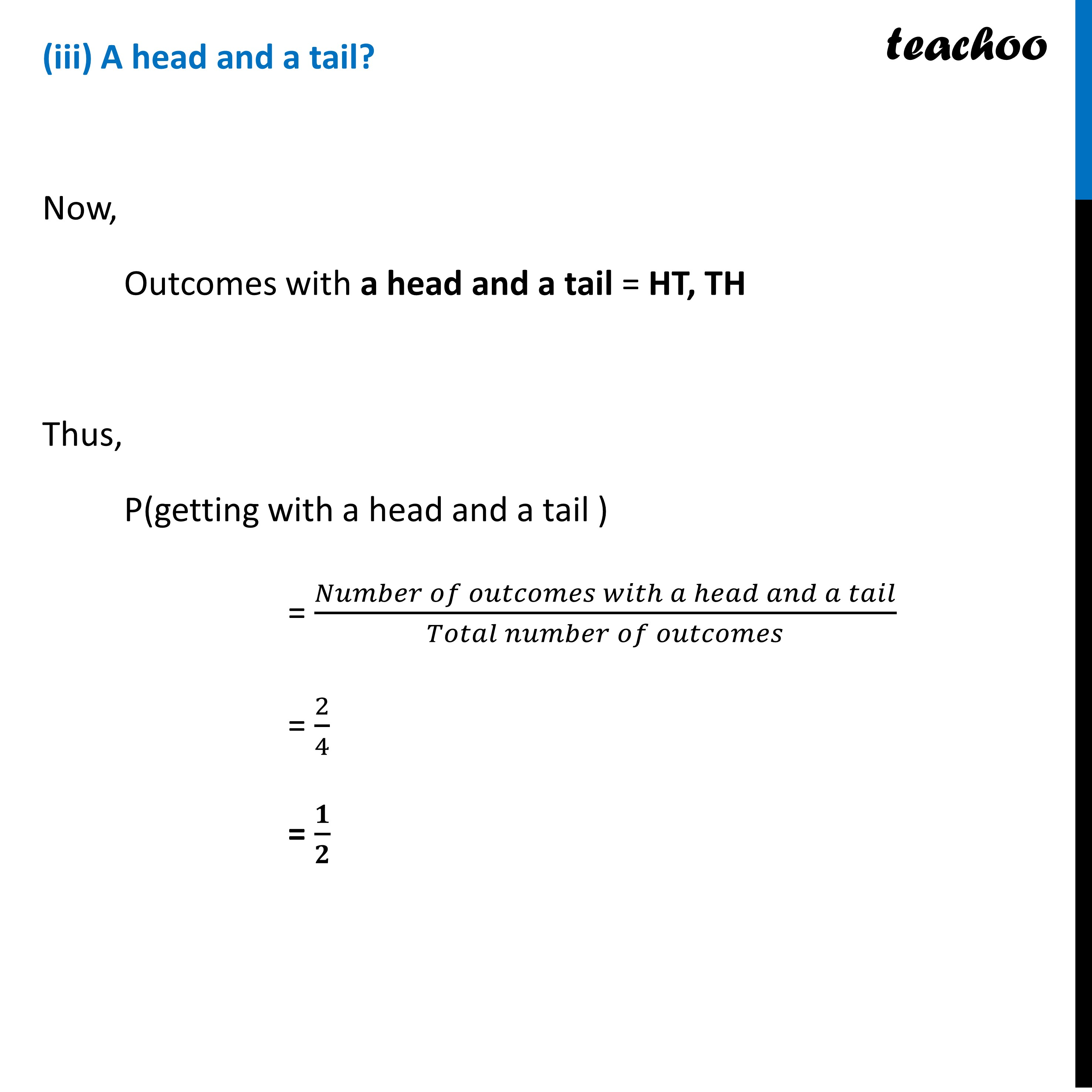CBSE Class 10 Sample Paper for 2023 Boards - Maths Standard

Class 10
Solutions of Sample Papers for Class 10 Boards

## (iii) A head and a tail?

This question is Similar to Question 6 - Past Year MCQ - Chapter 15 Class 10Learn in your speed, with individual attention - Teachoo Maths 1-on-1 Class

### Transcript

Question 31 Two coins are tossed simultaneously. What is the probability of getting (i) At least one head? (ii) At most one tail? (iii) A head and a tail?When 2 coins are tossed Possible outcomes = HH, TH, HT, TT Let’s solve each part individually (i) At least one head? Now, Outcomes with atleast with 1 head = HH, HT, TH Thus, P(getting atleast 1 head) = (𝑁𝑢𝑚𝑏𝑒𝑟 𝑜𝑓 𝑜𝑢𝑡𝑐𝑜𝑚𝑒𝑠 𝑤𝑖𝑡ℎ 𝑎𝑡𝑙𝑒𝑎𝑠𝑡 1 ℎ𝑒𝑎𝑑)/(𝑇𝑜𝑡𝑎𝑙 𝑛𝑢𝑚𝑏𝑒𝑟 𝑜𝑓 𝑜𝑢𝑡𝑐𝑜𝑚𝑒𝑠) = 𝟑/𝟒(ii) At most one tail? Now, Outcomes with atmost with 1 tail = HH, HT, TH Thus, P(getting atmost 1 tail) = (𝑁𝑢𝑚𝑏𝑒𝑟 𝑜𝑓 𝑜𝑢𝑡𝑐𝑜𝑚𝑒𝑠 𝑤𝑖𝑡ℎ 𝑎𝑡𝑚𝑜𝑠𝑡 1 𝑡𝑎𝑖𝑙)/(𝑇𝑜𝑡𝑎𝑙 𝑛𝑢𝑚𝑏𝑒𝑟 𝑜𝑓 𝑜𝑢𝑡𝑐𝑜𝑚𝑒𝑠) = 𝟑/𝟒 (iii) A head and a tail? Now, Outcomes with a head and a tail = HT, TH Thus, P(getting with a head and a tail ) = (𝑁𝑢𝑚𝑏𝑒𝑟 𝑜𝑓 𝑜𝑢𝑡𝑐𝑜𝑚𝑒𝑠 𝑤𝑖𝑡ℎ 𝑎 ℎ𝑒𝑎𝑑 𝑎𝑛𝑑 𝑎 𝑡𝑎𝑖𝑙)/(𝑇𝑜𝑡𝑎𝑙 𝑛𝑢𝑚𝑏𝑒𝑟 𝑜𝑓 𝑜𝑢𝑡𝑐𝑜𝑚𝑒𝑠) = 2/4 = 𝟏/𝟐